# Time Duration Calculator: Elapsed Time

The time difference (time elapsed) between two times may be calculated using this time duration calculator. It is as simple as adding "Start Time" and "End Time" and pressing the "calculate" button to compute the time elapsed instantly. This calculator also handles time intervals that include midnight properly.

1. Use Start From Text Box to Enter Starting Month, Year, Date, Hours, Minutes and seconds.
2. Use End At Text Box to Enter Ending Month, Year, Date, Hours, Minutes and seconds.
3. Click Calculate Button to get the duration between time and dates.
4. See the results in Drop Down window Box.
5. Use Clear Button to clear All the Time entered.
6. Use Print Button To Print the complete Results.
7. Use Email Box if you want to recieve the complete Time Duration Results at your Email ID.About the Author Nauman Rehmat Nauman is a Digital Marketing Specialist and owner of several online tools like DrEmployee. He believes in helping common people by providing a free online solution to day to day tasks. This project is one of them to offer free financial tools and tips.

## How to use Time Duration Calculator(Time Elapsed)?

Calculating the Time Elapsed (duration) between two times and dates can be a tricky job, especially when you dealing with times of two different dates, where start time value could be higher than the end time value, confusing, that how to subtract them. However, if you use the right tool for such calculations, then you can save a lot of time and energy and get an accurate result.

Keeping the things as mentioned earlier in mind, our developers have designed and created a state-of-the-art calculator, that is simple, compact, yet accurate enough to calculate the elapsed time within the fraction of time.

Following is the step by step guide to using our Time Elapsed calculator most efficiently: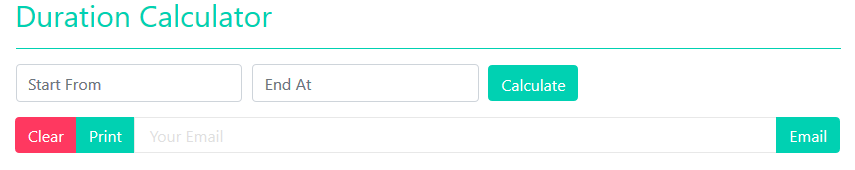Upon launching the calculator, you will see a form having a "Start From" and "End At" fields along with some other important buttons, as shown in the picture above.

You can use our calculator in many ways according to your need, however, below are most commonly used three methods to use your calculator effectively:

### Method 1: Calculating duration between two times (on the same date):

If you want to calculate Time elapsed between two times, then follow the steps below:

Step 1: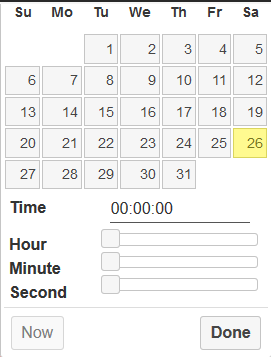1. Click on "Start From" Textbox, to launch a menu as shown in the image below:
1. Here, you have a calendar to select a date. (which you will keep unchanged for this method). Next, you will have 3 Horizontal scroll bars, 1st for Hours, 2nd for minutes, and last for selecting the seconds.
2. Choose your Start Time, using these scrollers, and click on the "Done" button.

Note: You can also use the "Now" button to select the current time and date.

Step 2:

1. Next, you need to input your "End Time" Click on the "End At" box to launch another menu similar to the one in step one.
2. Follow Point 2 and 3 of Step 1 to choose your End Time Hours, Minutes, and Seconds.
3. Once you have selected your Start and End Time, click on the "calculate" button to reveal the results.

### Method 2: Calculating duration (number of days) between two dates:

1. To calculate the number of days between two dates, you need to click on the "Start From" box and select only your Start Date and press the "Done" button.
2. Next, click on the "End At" box and select only your End Date and press the "Done" button.
3. Finally, click on the "Calculate" button to determine the number of Days between your defined dates.

### Method 3: Calculating duration between two times on different dates:

The calculating period between two times, occurring on different dates, is one of the complicated and confusing things if done manually. Therefore, you are recommended to use our Time Elapsed Calculator by following the method below for accurate result:

Step 1:

1. Click on "Start From" Textbox, to launch a menu as shown in the image below:1. Here, you have to select your start date from the calendar. Next, you will have 3 Horizontal scroll bars, 1st for Hours, 2nd for minutes, and last for selecting the seconds.
2. Choose your Start Time, using these scrollers, and click on the "Done" button.

Note: You can also use the "Now" button to select the current time and date.

Step 2:

1. Next, you need to input your "End Time and Date" for which click on the "End At" box to launch another menu similar to the one in step one.
2. Follow Point 2 and 3 of Step 1 to choose your End Time Date, Hours, Minutes, and Seconds.
3. Once you have selected your Start and End Time and Dates, click on the "calculate" button to reveal the results.

Result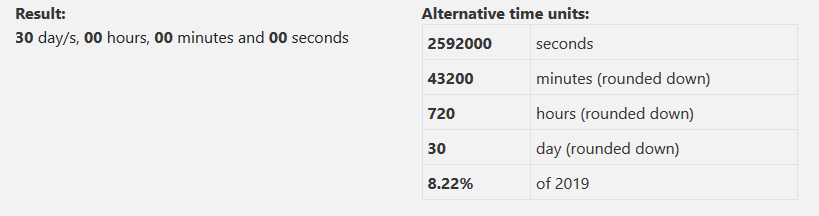• The result revealed from all three methods discussed above would look like the example image above.
• Here the number of Days as well as Hours, Minutes, and Seconds between your defined times would be displayed on the left side.
• As an Additional Information, a table is also displayed on the right side that converts the result to Decimal Seconds, Decimal Minutes, Decimal Hours, Decimal Days, and the percentage of Time (result) with respect to the number of days in the year.
• If you want to reset the calculator, click on the "Clear" button to start all over again.
• You also have an option to print the result by clicking on "Print" Button. Moreover, you can also send the outcome via email by entering the recipient's email address in "Your Email" textbox and clicking on the "Email" button.

Quick Tip:

You can easily embed our calculator on your website, by clicking on "Embed this calculator"  to reveal the embed code. Copy the code and paste it on your website.

## FAQs

#### Regular Formula:

A simple formula to calculate Elapsed Time is:

End TimeStart Time = Elapsed Time

#### Ms. Excel Formula:

Ms. Excel Formula to calculate Elapsed Time is explained by an example below: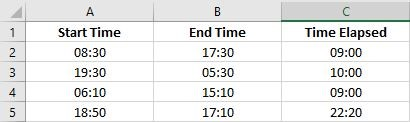• Although the basic formula is to subtract the start time from end time, which you can also use on Ms. Excel. As in the above image, you can easily subtract Cell B2 from Cell A2 to get accurate Time elapsed in Cell C2, but when using Ms. Excel, you have to be quite specific with how to use the formula to get precise results. In the image above, you can see that there are times when the value of Start Time is higher than End Time, so if we subtract this start time from end time using the basic formula, the Time Elapsed calculated by Ms. Excel would be wrong due to negative value.

To solve this problem, the perfect formula and procedure are the following:

1. Make sure all the values of Time from Cell A2 till Cell C5 are in the same time format. Here we have used the Custom time format by:
1. Selecting Cells from A2 till C5, and navigating to Home tab > Number Section > Selected "More Number Formats" from the Dropdown menu > Selected "custom" from Category List.
2. Here we typed our custom format hh:mm under "Type:" Label.
2. Now enter the formula =IF(B2 < A2, 1 + B2 - A2, B2- A2) in cell
3. After results are displayed in cell C2, select the Cell and click on the fill handle and drag it downwards till cell C5, to automatically copy and paste the formula to the rest of the cells for Time Elapsed Calculation. This formula no applies the condition to eliminate the possibility of negative value if Start Time is higher than End Time.

Answer: Elapsed Time is simply a duration of an event. In other words, it is the difference between the Start time and End Time of an Event.

Solution:

To calculate Elapsed Time between 10:50 AM and 3:45 PM, use any one of the following methods:

#### Method 1: Manual Calculation

1. As 10 50 AM comes before 3 45 PM in a day, we would take 10:50 PM as Start Time and 3:45 PM as End Time of an Event.
2. To avoid chances of human error, you are recommended to convert both of your times into Military Time by:
1. As in Regular Time and Military Time, the first 12 Hours of the Day are written the same, so at 10:50 AM, we will remove AM to convert it into Military Time (24-Hours Format). Military Time: 10 50
2. For 3:45 PM, we will add 12 Hours in regular Hours, remove PM and keep the minutes unchanged to get Military Time: 15 45
3. Now subtract Start Time from End Time to get Elapsed Time by:
1. Start by subtracting Minutes. In our case, 45 Minutes of End Time is less than 50 Minutes of Start Time, so you will get a negative value, which is not acceptable in Time. For which Add 60 minutes in End Time and subtract 1 hour from original hours of End Time to make its value higher than the Start Time.

NEW End Time Minutes: (45+60) = 105 minutes, NEW End Time Hours: (15-1) = 14 Hours

1. Now, subtract the Start Time Minutes from New End Time Minutes. (105-50) = 55 Minutes
2. Now Subtract the Start Time Hours from New End Time Hours: (14-10) = 4 Hours

Your Time Elapsed is 4 Hours and 55 Minutes.

#### Method 2: Instant Calculation

Feeling confused after reading method 1, this was to calculate ONE time Elapsed, what if you want to calculate 10 or 20 Time Elapsed at one go? It will take more than an hour to perform such calculations manually. Don’t Worry! Use our Military Time Calculator and Time Elapsed Calculator to get this work done in a Minute.

Note: As 10:50 AM is earlier than 3:45 PM, we would take 10:50 AM as Start Time of an event and 3:45 PM as End time of an Event, assuming that the event took place and ended on the same day.

To calculate Elapsed Time between 10:50 AM and 3:45 PM, first convert both of these times into Military Time using our Military Time Converter. (Result is shown in the images below):

##### Step 1:

10:50 AM conversion: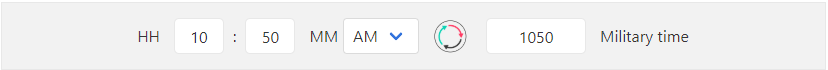##### Step 2:

3:45 PM conversion: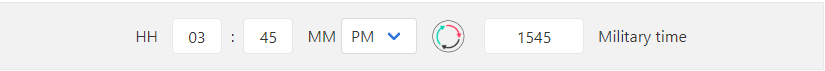##### Step 3:

Now you have Start Time: 1050 and End Time: 15 45, Select Start Time from Start From menu and End Time from End At menu on our Time Elapsed Calculator, located at the top of this page. See the image below as a worked example: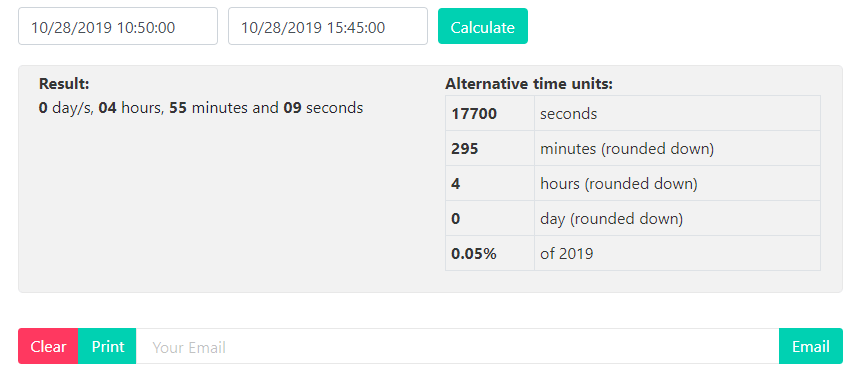Here you get the Time Elapsed as 4 Hours and 55 Minutes, Just in 3 Simple Steps.

Solution: There are several methods to calculate the Time elapsed; 2 of them are mentioned in FAQs Question # 3. Here is the answer to this question, using method 1 of FAQs Question # 3:

Note: As 5:45 AM, it comes earlier than 4:10 PM in a Day, so we would take 5:45 AM as Start Time and 4:10 PM as End Time of an Event (Assuming that the event started and ended on the same day).

To calculate Time Elapsed subtract Start Time from End Time by:

1. Convert Both Start Time and End Time into 24 Hours (Military Time) using method explained in FAQs Question # 3 – Method 1 OR using our Military Time Converter to get

Start Time: 05 45 and End Time: 16 10

1. As Minutes of End Time is less than Minutes of Start Time so add 60 Minutes to Original Minutes of End Time and Subtract 1 Hour from Original Hours of End Time to get End Time: 15 Hours 70 Minutes.
2. Now subtract Start Time Minutes from End Time Minutes: 70 – 45 = 25 Minutes.
3. Subtract Start Time Hours from End Time Hours: 15-5 = 10 Hours.
4. Your Time Elapsed is 10 Hours and 25 Minutes.

Answer: There are several Formulas for Time; here are some of the most relevant one:

• add Hours of both times.
• Add Minutes of both Times
• If the minutes are more than 60, subtract 60 minutes from the Minutes and add 1 hour to Hours.

Quick Tip: Use our Hour Calculator to get this calculation done instantly.

#### Subtracting Time:

To subtract Time, follow these steps:

• Subtract the Hours.
• Subtract the Minutes.
• If the total value of Minutes is Less than zero (negative), then add 60 minutes in Minutes and subtract 1 Hour from Hours.

Quick Tip: Use our Time Elapsed Calculator located at the top of this page to get this work done instantly.

Answer: There are numerous ways to calculate Time, but the most appropriate method to this website is explained correctly in FAQs Question # 5.

Answer: Elapsed Flying time is a time an airplane spends in the air, excluding stopover time and Time taxiing to the gate and from the gate.

Answer: To determine the number of weeks between two dates, follow the steps below:

1. Select your Start Date from the Start From box and End Date from End At box on our Time Elapsed Calculator.
2. Once selected both dates, click on the "Calculate" button to determine the number of days between your defined dates.
3. Lastly, divide the number of days between your defined dates with 7 to calculate the number of weeks.
Other Calcutors